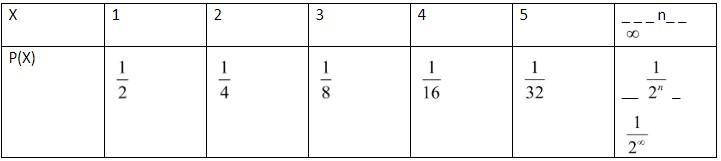QuestionAnswers

# A coin is tossed repeatedly until it shows its head. Let X be the number of tosses required to get head. Write the probability distribution of X.

Hint: In this problem, we will use the concept of conditional probability to write the probability distribution of X. If A and B are two events in a sample space S, then the conditional probability of A, with B given is defined as:

$P\left( {}^{A}/{}_{B} \right)=\dfrac{P\left( A\cap B \right)}{P\left( B \right)}$

Conditional probability is a measure of the probability of an event occurring given that another event has (by assumption, presumption, assertion or evidence) occurred. If the event of interest is A and the event B is known or assumed to have occurred, “the conditional probability of A given B”, or “ the probability of A under the condition B”, is usually written as $P\left( {}^{A}/{}_{B} \right)$.

This may be visualized as restricting the sample space to situations in which B occurs. The logic behind this equation is that if possible outcomes for A and b are restricted to those in which B occurs, this set serves as the new sample space.

When A and B are independent events, then:
$P\left( {}^{A}/{}_{B} \right)=P\left( A \right)\,\,\,\,and\,P\left( {}^{B}/{}_{A} \right)=P\left( B \right)............(1)$

When we flip a coin, there are two possible outcomes: heads and tails. Each outcome has a fixed probability, the same from trial to trial. In the case of coins, heads and tails each have the same probability of $\dfrac{1}{2}$.
In this question, it is given that a head should be obtained.
If we flip the coin once, then the probability of getting head is $\dfrac{1}{2}$.

But, if we don’t get head in one flip, then the probability of getting head in the second flip given as P(E) is:
\begin{align} & P\left( E \right)=P\left( A \right).P\left( {}^{B}/{}_{A} \right) \\ & \,\,\,\,\,\,\,\,\,\,\,\,\,=P\left( A \right).P\left( B \right) \\ \end{align} ( from equation 1 )

Where P(A) and P(B) are the probability of getting tails and heads respectively.
So, the probability distribution of X is given as:Note: Students should note that here A and B both are independent events. The occurrence of one event does not depend on the other. We can use equation (1) while solving the problem only when A and B are independent events.